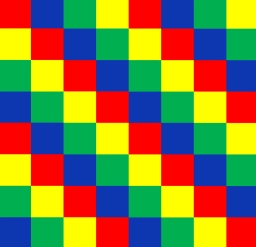# Evaluate 37

Evaluate the function K(x) = 2x²+3x-2 what is the value of K(½)

y =  0

### Step-by-step explanation:Did you find an error or inaccuracy? Feel free to write us. Thank you!

Tips for related online calculators
Are you looking for help with calculating roots of a quadratic equation?
Need help calculating sum, simplifying, or multiplying fractions? Try our fraction calculator.